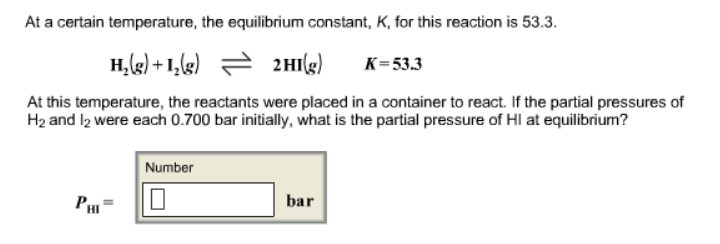# Problem: At a certain temperature, the equilibrium constant, K, for this reaction is 53.3. H2 (s) + I2 (g) ⇌ 2HI (g)           K = 533 At this temperature, the reactants were placed in a container to react. If the partial pressures of H2 and I2 were each 0.700 bar initially, what is the partial pressure of HI at equilibrium?

###### FREE Expert Solution
80% (400 ratings)###### Problem Details

At a certain temperature, the equilibrium constant, K, for this reaction is 53.3.

H2 (s) + I2 (g) ⇌ 2HI (g)           K = 533

At this temperature, the reactants were placed in a container to react. If the partial pressures of H2 and I2 were each 0.700 bar initially, what is the partial pressure of HI at equilibrium?